# Low Side Switch Design Using NMOS with Resistive Load

There are only few steps to consider in dealing with low side switch design using NMOS with a resistive load. Low side switch is the term used because once the switch turns on; it will connect the load to the circuit ground to complete the current path. A load is not limited to resistors, it can also an inductive such as relay and lamp. In this article we will concentrate with the resistive load.

### Low Side Switch Design Using NMOS Circuit Schematic

Figure 1 below is the circuit schematic of the low side switch we want to design. Rlimit is not necessary all the time. It is a must when using a power MOSFET in switching converter. Power MOSFET has high input capacitance. During startup this capacitance act as a short circuit so the initial peak current  is huge and may damage the device that drive the gate of the MOSFET. Another reason of adding this Rlimit to power MOSFET is to minimize the dv/dt. In small signal MOSFET, this is not needed.

In using power MOSFET in switching converters, the value of Rlimit is can be set according to the recommendation of the MOSFET vendor. This is usually less than 100Ω.

R1 is a must to include. This will ensure that MOSFET will stay off when the input voltage is removed. R1 is can be set to 10kΩ or higher.

For NMOS, the gate voltage must be higher than the source. The difference must be higher than the gate threshold voltage.

In above circuit, Vin is can be coming from a microcontroller or digital signal controllers/processors if the MOSFET is small signal type. If the MOSFET is power type that is use to switch on and off a switching converter, Vin cannot be directly a microcontroller or digital signal processors due to huge turn on current caused by higher input capacitance.

Below are the steps of Low Side Switch Design Using NMOS.

### Step 1: Select a MOSFET

We can start the low side switch design using NMOS by selecting a MOSFET. In selecting a MOSFET you need to know the load current that will flow to the MOSFET. Compare this load current to the drain current rating of the MOSFET. If the estimated load current is 500mA, better use a MOSFET with a 1A capability to provide margin. A 1 ampere rated MOSFET is not anymore 1A when the ambient temperature is higher than 25’C. Of course, the MOSFET must be an N channel or NMOS.

Select a MOSFET that has a drain voltage capability higher than the maximum level of Vdd in the circuit. As a rule of thumb, utilize only up to 70%. Say the maximum voltage the device can handle is 30V, the maximum level of Vdd must only be 21V.

Also consider the gate threshold voltage in the selection of the device. If you are going to drive the MOSFET gate with a microcontroller or digital ICs, the maximum gate threshold voltage of the MOSFET must be less than 5V (Vcc level of the microcontroller) with a margin.

### Step 2: Satisfy the VGS Threshold Requirement

In low side switch design using NMOS, the gate threshold voltage must be satisfied. The applied gate to source voltage must be positive unlike PMOS which requires a negative gate to source voltage. In order to fully saturate an NMOS, its applied gate to source voltage must be significantly higher than the threshold level. For 2N7002 part, the maximum threshold voltage needed is 2.75V based on below table, but do not just apply same level into the gate of the above circuit. If we are going to use a microcontroller in the Vin, the applied gate to source voltage will be 5V (MCU has 5V usual output).To turn off the MOSFET, the level of Vin in above schematic must be less than the minimum threshold voltage in the table above. If you notice in the table above, there are different values for the minimum threshold. These are taken at different conditions. In order to cover everything, you may consider the minimum of them all.

### Step 3: Check the Drain Current

With the selected MOSFET and the load, check the drain current using below equation.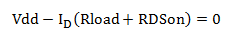RDSon is the on-state resistance of the MOSFET. Supposing we are using 2N7002 part, the RDSon is 3.8Ω to 5.3Ω taken at 25’C for VGS of 4.5V.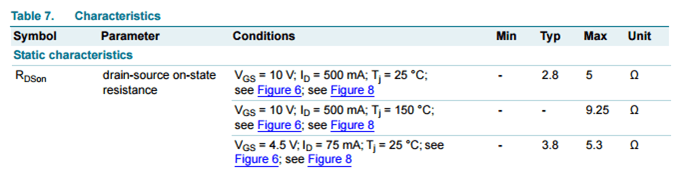If the circuit is exposed to ambient temperatures higher than 25’C, the RDSon may increase. In this case, we will get the relative value on the graph below.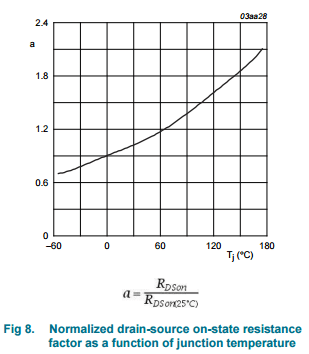Supposing the maximum temperature the circuit is exposed to is 60’C, the resulting RDSon isIn checking the drain current our objective is to know the device stress. Thus, we can use the minimum value of the RDSon (or make it zero) to get the worst case. Considering the value of Rload is 100Ω and the level of Vdd is 10V, the drain current is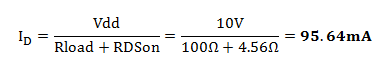The given drain current in the datasheet of 2N7002 is 300mA maximum at 25’C.Above drain current rating is only a typical value. At 60’C operation, this drain current will de-rate to around 80% based from below graph.So the actual current rating of the device at 60’C operating temperature isThe computed drain current is less than the capability of the device; the MOSFET is safe in terms of current stress.

### Alternate Approach to Compute the Drain Current Rating of the Device

In case the temperature versus drain current graph is not available, you can use below equation to solve for the drain current capability of the MOSFET.

For Small Signal MOSFETFor Power MOSFETWhere;

Tjmax is the maximum junction temperature of the MOSFET, Tamax is the maximum operating ambient temperature, Tcmax is the maximum case temperature of the MOSFET in actual use, Rthja is the thermal resistance from junction to ambient, Rthjc is the thermal resistance from junction to case and RDSon is the on state resistance.

We will apply above equation in 2N7002 part. 2N7002 is a small signal MOSFET so we are going to use the first equation. The Tjmax is 150’C, the Rthja is 350K/W and our computed RDSon for 60’C operating ambient is 4.56Ω typical.As you can see, the result is close to the value given by the graph above. You can also use the computed maximum RDSon above to get the worst case drain current capability.

### Step 4: Check the Power Dissipation

Use below equation to check the power capability of the device and compare this to the computed power dissipation.For 2N7002,The power dissipated  by the MOSFET is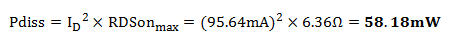The computed power dissipation is less than the capability of the device with a huge margin. Therefore there is no danger related to power stress.

This site uses Akismet to reduce spam. Learn how your comment data is processed.